### Home > INT3 > Chapter 8 > Lesson 8.2.2 > Problem8-78

8-78.
1. For each of the following sets of numbers, what is the equation of a function that has these numbers as roots? Homework Help ✎

1. –3 + i and –3 – i

2. 5 +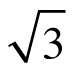and 5 –3. –2,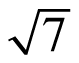, and –4. 4, –3 + i, and –3 – i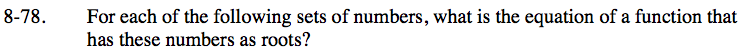Refer to problems 8-65 and 8-67.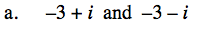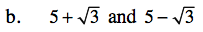y = x2 − 10x + 22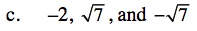You will need to write a product of three linear factors for this one.

y = x3 + 2x2 − 7x − 14You can use your answer from part (a) to get started.